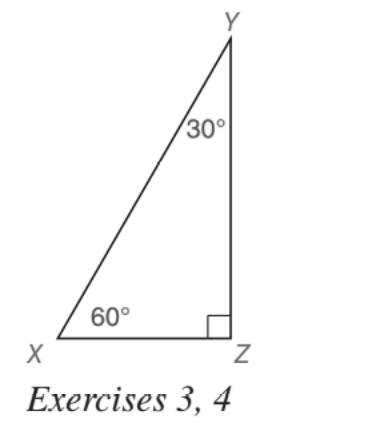Chapter 5.5, Problem 3EElementary Geometry For College St...

7th Edition
Alexander + 2 others
ISBN: 9781337614085

Solutions

Chapter
SectionElementary Geometry For College St...

7th Edition
Alexander + 2 others
ISBN: 9781337614085
Textbook Problem

For the 30 ° - 60 ° - 90 ° triangle shown, suppose that X Z = a . Find: a) Y Z b) X YTo determine

a) To find:

YZ, for the 30°-60°-90° triangle shown, suppose that XZ=a.

Explanation

Approach:

For a right triangle, for which the measure of the interior angles 30°, 60°, and 90°; if ‘a’ is the length of measure of the shorter leg; opposite to the angle 30°, then the length of the other two sides is given by

Length of the longer leg (opposite to 60°) =a3

Length of the hypotenuse (opposite to 90°)=2a.

In general

Length of the longer leg =3× (Length of the shorter leg)

Length of the hypotenuse =2× (Length of the shorter leg)

Calculation:

Given,

A 30°-60°-90° triangle XYZ with XZ=a.

30°-60°-90° theorem

To determine

b) To find:

XY, for the 30°-60°-90° triangle shown, suppose that XZ=a.

Still sussing out bartleby?

Check out a sample textbook solution.

See a sample solution

The Solution to Your Study Problems

Bartleby provides explanations to thousands of textbook problems written by our experts, many with advanced degrees!

Get Started

lf f(x) = x2 2x + 3, evaluate the difference quotient f(a+h)f(a)h

Single Variable Calculus: Early Transcendentals, Volume I

Evaluate the integral. 37. 0/4tan3sec2d

Single Variable Calculus: Early Transcendentals

True or False: is monotonic.

Study Guide for Stewart's Single Variable Calculus: Early Transcendentals, 8th